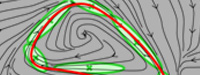### Abstract

We present a probabilistic approach to learning robust models of human motion through imitation. The combination of Hidden Markov Model (HMM) and Gaussian Mixture Regression (GMR) allows us to extract redundancies across multiple demonstrations and build time-independent models to reproduce the dynamics of the observed movements. The approach is first compared with state-of-the-art approaches by using generated trajectories sharing similar characteristics to those of humans. Three applications on different types of robots are then presented. An experiment with the iCub humanoid robot acquiring a bimanual dancing motion is first presented to show that the system can cope with cyclic and crossing motions. An experiment with a 7 DOFs WAM robotic arm learning the motion of hitting a ball with a table tennis racket is presented to highlight the possibility to encode several movements in a single model. Finally, an experiment with a HOAP-3 humanoid robot holding a spoon and learning to feed the Robota humanoid robot is presented. It shows the capability of the system to handle several constraints simultaneously.

### Bibtex reference

@article{Calinon10RAM,
author="Calinon, S. and D'halluin, F. and Sauser, E. L. and Caldwell, D. G. and Billard, A. G.",
title="Learning and reproduction of gestures by imitation: {A}n approach based on Hidden {M}arkov Model and {G}aussian Mixture Regression",
journal="{IEEE} Robotics and Automation Magazine",
publisher="IEEE",
year="2010",
volume="17",
number="2",
pages="44--54"
}

### Video

The experiment consists of learning and reproducing the motion of hitting a ball with a table tennis racket by using a Barrett WAM 7 DOFs robotic arm. One objective is to demonstrate that such movements can be transferred using the proposed approach, where the skill requires that the target be reached with a given velocity, direction and amplitude.

This experiment aims at demonstrating that the framework can be used in an unsupervised learning manner, i.e. where several movements can be encoded in a single HMM, without specifying a priori the number of movements, and without having to associate the different motions with a class or label.

### Source codes

#### DownloadDownload GMR Dynamics sourcecode

#### Usage

Unzip the file and run 'demo1' in Matlab.

#### Reference

• Calinon, S., D'halluin, F., Sauser, E.L., Caldwell, D.G. and Billard, A.G. (2009) A probabilistic approach based on dynamical systems to learn and reproduce gestures by imitation. IEEE Robotics and Automation Magazine.

#### Demo 1 - Demonstration of a trajectory learning system robust to perturbation based on Gaussian Mixture Regression (GMR)This program first encodes a trajectory represented through time 't', position 'x' and velocity 'dx' in a joint distribution P(t,x,dx) through Gaussian Mixture Model (GMM) by using Expectation-Maximization (EM) algorithm. Gaussian Mixture Regression (GMR) is then used to estimate P(x,dx|t), which retrieves another GMM refining the joint distribution model of position and velocity.
The learned skill can then be reproduced by combining an estimation of P(dx|x) with an attractor to the demonstrated trajectories.